# How to use the VDB Function in Excel

VDB function is Variable declining balance depreciation method is the way of calculating the depreciation of any asset within a specific period. “Variable declining balance” method is the way where the depreciation of an asset can be calculated for any interval of period.
The VDB function returns depreciation decline amount within any interval of period.

Syntax:

=VDB (cost, salvage, life, start_period, end_period, [factor], [no_switch])

cost : Cost price of the asset
salvage : remaining price of the asset after its lifetime period
life : period after which cost price becomes salvage price
start_period : start year period for the depreciation
end_period : end year period for the depreciation
[factor] : [optional] factor or rate at which depreciation declines. Default is set to 2.
[no_switch] : [optional] default is False. It is Switch when the depreciation is greater than the declining balance calculation, it doesn’t switch to straight line depreciation.
Let’s understand this function using it in an example.

Here we took a product as Iphone. The cost price & salvage price of the latest Iphone is shown in the snapshot below.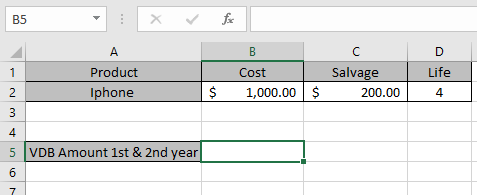Now we need to calculate the depreciation amount for the Iphone within 1st year & 2nd year period.

Use the formula to calculate depreciation:

=VDB (B2, C2, D2, 1, 2)

B2 : Cost price of the asset
C2 : remaining price of the asset after a period
D2 : 4 years after cost price becomes salvage price
1 : start year to calculate depreciation amount.
2 : end year to calculate depreciation amount.
Factor : default is 2
Switch : default is False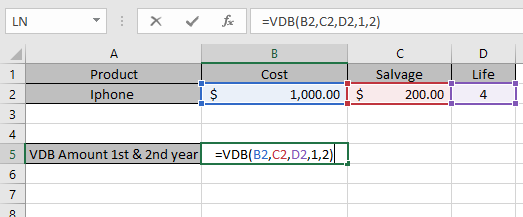The values to the formula are taken as cell reference.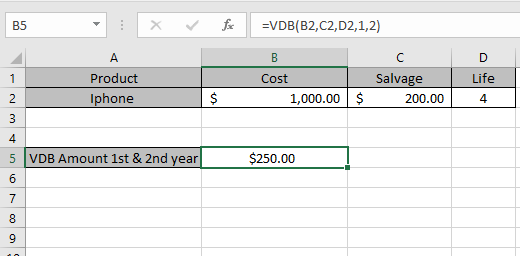As you can see from the above returned value, within first year & second year \$ 250 will depreciated from the asset cost value.
Now we will calculate the depreciation amount for different year intervals separately.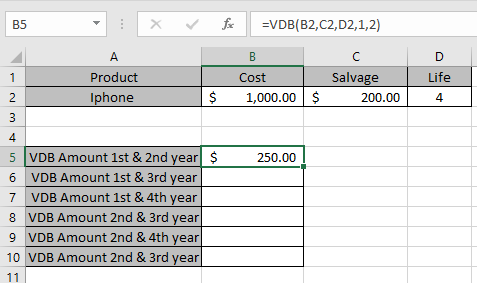Use the formula to calculate depreciation within 1st year & 3rd year :

=VDB (B2, C2, D2, 1, 3)

B2 : Cost price of the asset
C2 : remaining price of the asset after a period
D2 : 4 years after cost price becomes salvage price
1 : start year to calculate depreciation amount.
3 : end year to calculate depreciation amount.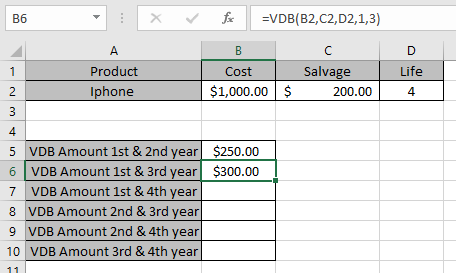Similarly calculate the depreciation for all others.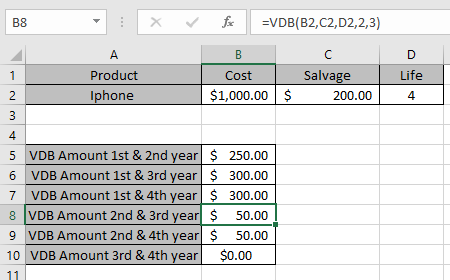So, we got the variable declining balance depreciation for each period

 Start year period End year period Depreciation 1 2 \$ 250.00 1 3 \$ 300.00 1 4 \$ 300.00 2 3 \$ 50.00 2 4 \$ 50.00 3 4 \$ 00.00

This is the declining depreciation table for the asset.

Note:

1. The function generates #VALUE! Error if arguments to the function is non - numeric except optional switch.
2. The function generates #NUM! Error if:
• The value of cost and salvage is less than 0.
• The value of life of asset is less than or equals to zero.
• The period argument is less than or equals to 0 or greater than life period.
• Start period greater than end period.
1. Switch function checks if depreciation is greater than the declining balance calculation and :
1. If Switch is True, the function doesn’t switch to straight line depreciation
2. If Switch is False or default, the function switches to straight line depreciation.

Hope you understood how to use VDB function and referring cell in Excel. Explore more articles on Excel  financial functions here. Please feel free to state your query or feedback for the above article.

Related Articles:

How to use the DB Function in Excel

How to use the DDB Function in Excel

How to use the SYD Function in Excel

Popular Articles:

50 Excel Shortcuts to Increase Your Productivity

How to use the VLOOKUP Function in Excel

How to use the COUNTIF in Excel 2016

How to use the SUMIF Function in Excel

Terms and Conditions of use

The applications/code on this site are distributed as is and without warranties or liability. In no event shall the owner of the copyrights, or the authors of the applications/code be liable for any loss of profit, any problems or any damage resulting from the use or evaluation of the applications/code.